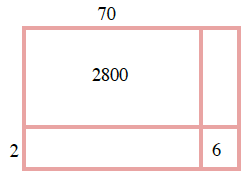### Home > CC1 > Chapter Ch2 > Lesson 2.3.2 > Problem2-65

2-65.

Draw a generic rectangle to help you calculate each product. For each part, write: (total dimension)(total dimension) = sum of individual area parts = total area. For example, the diagram at right shows that $(12)(27)=200+40+70+14=324$. Homework Help ✎

1. $( 73 ) ( 42 )$

• Think back to the way you solved the generic rectangles in class. Change 73 to 70 + 3. Change 42 to 40 + 2.

• Part of the rectangle has been filled in for you.

•1. $125 \cdot 81$

• This one is solved just like the one above. Remember to add a hundreds place column.

 $10$ $+2$ $20$ $200$ $40$ $+7$ $70$ $14$